### Home > AC > Chapter 14 > Lesson 14.3.1.5 > Problem3-66

3-66.
1. Jack and Jill were working on simplifying the expression below, but they were having some trouble. Then Jill had an idea. Homework Help ✎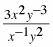“Can't we separate the parts?” she said. “That way, it might be easier to tell what we can simplify.” She rewrote the expression as shown below.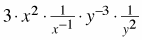“Okay,” said Jack. “Now we can rewrite each of the parts with negative exponents and simplify.”

1. Help Jack and Jill finish simplifying their expression.

2. Use their idea to rewrite and simplify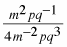.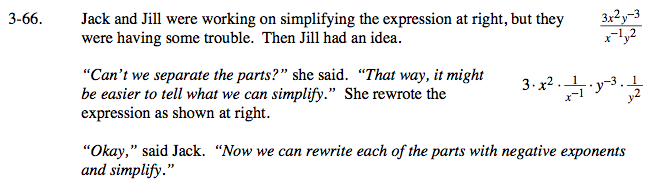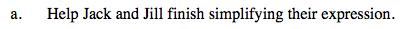Notice that Jill used the Associative Property and put the factors with the same variables next to each other.

$\text{Remember, }\frac{1}{x^{-1}}=x.\text{ What does }y^{-3}\text{ equal}?$

$\frac{3x^3}{y^5}$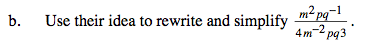$\frac{1}{4}\cdot m^2\cdot\frac{1}{m^{-2}}\cdot p\cdot\frac{1}{p}\cdot q^{-1}\cdot\frac{1}{q^3}$